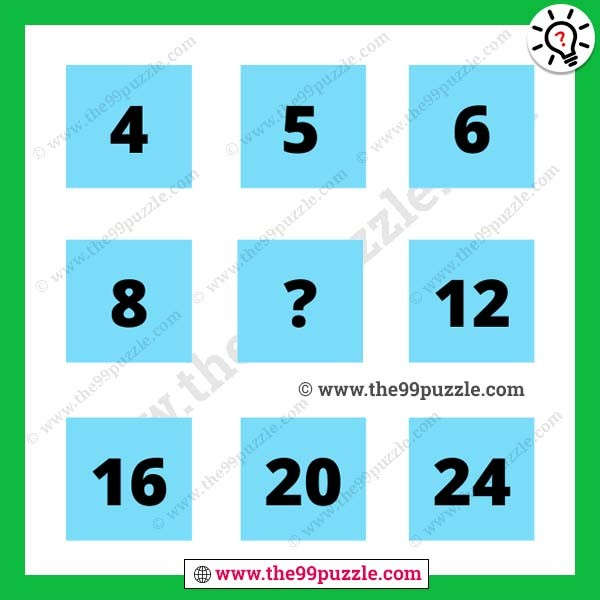# Challenging math brain teaser for adults – Puzz245

In this challenging math brain teaser for adults. Can you solve this box puzzle? These types of puzzles are most important in competitive exams and interviews. This is the one type of most popular puzzle. Missing number puzzles are interesting and fun math. Whenever you try to solve these math puzzles, this gets very hard. But sometimes these puzzles can solve you within a second. In this tricky math puzzle, you get some numbers within the box. You have to crack the math logic and replace the question mark.###### Explanation:

1st row = 4 (+1) → 5 (+1) → 6

2nd row = 8 (+2) → 10 (+2) → 12

3rd row = 16 (+4) → 20 (+4) → 24